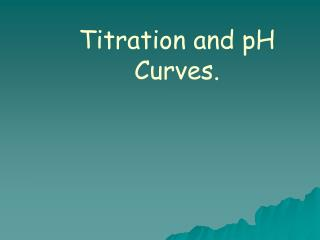# Titration and pH Curves. - PowerPoint PPT PresentationDownload PresentationTitration and pH Curves.

Titration and pH Curves.
Download Presentation## Titration and pH Curves.

- - - - - - - - - - - - - - - - - - - - - - - - - - - E N D - - - - - - - - - - - - - - - - - - - - - - - - - - -
##### Presentation Transcript

1. Titration and pH Curves.

2. Titration and pH Curves. A titration curve is a plot of pH vs. volume of added titrant.

3. Parts of a titration Titrant- acid or base of known concentration that is added to the substance being analyzed. Analyte- the substance that is being analyzed, or your unknown.

4. Titrations Because titrations involve small concentrations, and mL are of often used in titrations, and millimoles, or mmol. Molarity = mmol/mL The equivalence point is when moles of titrant are equal to moles of analyte. All volumes in a titration are considered to be additive. Always label the equivalence point and for weak acid or base titrations the half equivalence point, where pH = pKa.

5. Strong Acid-Strong Base Titration Curves. Before the addition. pH is calculated directly from the initial concentration. Additions before the equivalence point. Construct a “stoichiometry” reaction table. Determine MOLES of acid in excess (not neutralized). Divide MOLES by the TOTAL VOLUME to obtain [H3O+]. Calculate the pH.

6. Strong Acid-Strong Base Titration Curves. Additions at the equivalence point. The pH ALWAYS is equal to 7.00 when [H3O+] = [OH-]. Additions beyond the equivalence point. Construct a “stoichiometry” reaction table.Determine MOLES of base in excess (not neutralized).  Divide MOLES by the TOTAL VOLUME to obtain [OH-].  Calculate the pOH, then the pH.

7. Problem 50.0 mL of 0.200 M HNO3 are titrated with 0.100 M NaOH. Calculate the pH after the additions of 0.0, 10, 20, 50, 100,150, and 200 mL samples of NaOH. Then, construct a titration curve and labelit properly.

8. Strong Acid Strong Base Problem 50.0 mL of 0.50 M HCl are titrated with 0.250 M NaOH. Calculate the pH after the additions of 0.0, 10, 20, 50, 100,150, and 200 mL samples of NaOH. Then, construct a titration curve and labelit properly.

9. Weak Acid-Strong Base Titration Curves. Before the addition. Construct an “equilibrium” reaction table ONLY! Ka = [A-] [H3O+] to obtain [H3O+]. [HA]  Calculate the pH.

10. Weak Acid-Strong Base Titration Curves. Additions before the equivalence point. Construct a stoichiometry reaction table. Determine MOLES of acid in excess (not neutralized) andMOLES of conjugate base formed. Divide MOLES by the TOTAL VOLUME to obtain [H3O+] and [A-]. Construct an “equilibrium” reaction table. Ka = [A-] [H3O+] and obtain [H3O+]. [HA]  Calculate the pH.

11. Half equivalence point The equivalence point is when the moles or titrant are equal to the moles of analyte. At half the equivalence point, exactly half of the weak analyte will be shifted into it’s conjugate. HA⇌ H+ + A-, therefore [HA] = [A-] pH = pKa + log [A-] / [HA] At the half equivalence point pH = pKa

12. Additions at the equivalence point. Construct a stoichiometry reaction table. Determine MOLES of conjugate base formed. Divide MOLES by the TOTAL VOLUME to obtain [A-]. Calculate Kb (Ka x Kb = Kw). Construct an “equilibrium” reaction table, reacting the conjugate base with water. Kb= [OH-] [BH+] and obtain [OH-]. [B] Calculate the pOH, then the pH. The equivalence point is ALWAYS >7!

13. Additions beyond the equivalence point Construct a “stoichiometry” reaction table. Determine MOLES of base in excess (not neutralized) and the MOLES of conjugate base. Divide MOLES by the TOTAL VOLUME, Because [OH-]excess >> [OH-]conj. base, use [OH-]excess to calculate pOH, then the pH.

14. Problem 50.0 mL of 0.10 M acetic acid (Ka = 1.8 x 10-5) are titrated with 0.10 M NaOH. Calculate the pH after the additions of 0, 10, 25, 40, 50, 60, and 75 mL samples of NaOH. Then, construct a titration curve and label it properly.